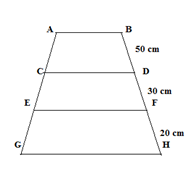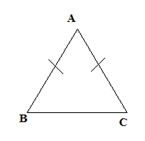# SSC CGL 2018 Practice Test Papers | Quantitative Aptitude (Day-16)

Dear Aspirants, Here we have given the Important SSC CGL Exam 2018 Practice Test Papers. Candidates those who are preparing for SSC CGL 2018 can practice these questions to get more confidence to Crack SSC CGL 2018 Examination.

[WpProQuiz 2888]

Click “Start Quiz” to attend these Questions and view Explanation

1) If  50% of (A+B) = 75% of (A-B) then what is the value of (2A-3B)/(A+B)?

a) 7/6

b) 2/3

c) 5/6

d) 3/2

2) One-fifth of 375 is what percent of 225?

a) 50

b) 66.66

c) 33.33

d) 12.5

3) What is 26% of 36% of 25/13 of 2100?

a) 478

b) 378

c) 388

d) 488

4) Ritu’s salary is 25% less than Geethu’s salary then Latter’s salary is how much percent greater than former’s salary?

a) 20

b) 25

c) 12.5

d) 33.33

5) In the given figure AB|| CD, CD||EF, EF||GH and AG=120 cm. Find the value of CE.a) 24 cm

b) 36 cm

c) 40 cm

d) 30 cm

6) In triangle ABC which two angles are equal?a) Angles A and B are equal

b) Angles C and B are equal

c) Angles A and C are equal

d) All the angles A, B and C are equal

7) A person makes 2 toys per hour while his son breaks 5 toys per day. Both works together for 20 hours a day then what is the number of dolls available for sale at the end of 2 days?

a) 50

b) 60

c) 70

d) 30

8) Cos (π+30)=x. What is the value of x?

a) -√3/2

b) √3/2

c) 0

d) 1

9) Cost Price of a motor bike and bicycle are in the ratio 5:1. Motorbike is marked 10 % above the cost price and bicycle is marked 20% above the marked price. Total selling price is 83750. What is the selling price of bicycle?

a) 16000

b) 15000

c) 19000

d) 20000

10) The range of reflex angle is

a) 0<θ<180

b) 0<θ<90

c) 180<θ<360

d) 180<θ<270

50/100 (A+B) = 75/100(A-B)

A=5B à(1)

Substitute (1) in (2A-3B) / (A+B)

7B/6B=7/6

1/5*375/225*100=33.33

26/100*36/100*25/13*2100 = 378

Let Rithu’s salary be 75 and Geethu’s salary be 100

25/75*100=33.33

AC: CE: EG = 5:3:2

CE = 120/10*3 = 36

In isosceles triangle angles opposite to the congruent sides are equal.

No. of dolls made by the person in a day= 2*20=40

No. of dolls his son break=5

No, of dolls available for sale= (40-5)*2=70

Cos (180+30)= − cos 30= − √3/2

Explanation:

Let the cost price of motor bike and bicycle be 5x and x respectively.

5x*110/100+x*120/100=83750

X=12500

Selling price of bicycle=12500*120/100=15000